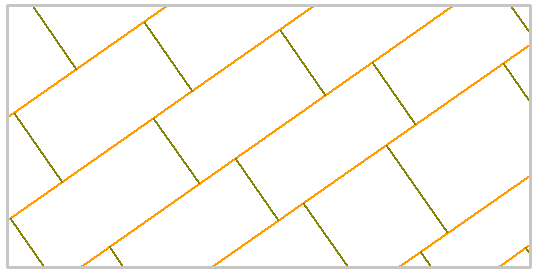# Joint Model: Cross Jointed

The Cross Jointed joint network model allows you to define a network which consists of two sets of parallel joints (e.g. bedding planes with cross joints) which intersect to form rectangular or trapezoidal blocks, as shown in the following figure. The spacing of the joint planes can be defined as random variables (i.e. assigned statistical distributions).

The bedding joint planes are assumed to be continuous (i.e. infinite), while the cross joints intersect the bedding planes at intervals defined by the spacing distribution. The cross joints do not have to be perpendicular to the bedding planes, but may intersect them at any angle.

Cross joint network, 35 degree bedding plane inclinationThe following input parameters can be defined for the Cross Jointed joint network model.

## Joint Property

For the Cross Jointed model, the bedding and cross joint properties can be specified independently by choosing the Bedding Joint Property and Cross Joint Property. This determines the physical properties (e.g. strength, stiffness) of the joints.

The joint property types are defined in the Define Joint Properties dialog.

## Orientation

The Orientation parameters for the Cross Jointed joint network model (Trace Plane, Inclination, etc) have the same definition as the Parallel Deterministic model, except that you must enter orientations for both bedding and cross joints. For details see the Parallel Deterministic topic.

NOTE:

• The bedding planes do not have to be horizontal, they may be at any orientation.
• The cross joints do NOT have to be perpendicular to the bedding planes. You can define any orientation for the cross joints, as long as they are not parallel to the bedding planes.
• For the Cross Jointed model, the joint orientations CANNOT be assigned statistical distributions. All bedding planes are assumed to be parallel, and all cross joints are assumed to be parallel, therefore the orientations are a constant value.
• For the Cross Jointed model, the Use Trace Plane option does NOT affect the definition of spacing, see below for details.

## Bedding Spacing

The Bedding Spacing is the perpendicular distance between the parallel bedding planes, as measured in the Trace Plane.

• To define the Bedding Spacing as a random variable, select a statistical distribution (Normal, Uniform, Exponential or Lognormal), and enter the mean, standard deviation and relative minimum and maximum values. See the Statistical Distributions topic for details about the statistical distribution parameters.
• To define the Bedding Spacing as a constant value, choose Distribution = None. In this case the spacing will be constant and equal to the mean value.

For the Cross Jointed model, it is important to note that the bedding spacing is ALWAYS measured in the Trace Plane. Therefore the value of bedding spacing which you enter, is the apparent 2-dimensional spacing. In general this will not be equal to the true 3-dimensional spacing, unless the bedding planes are perpendicular to the Trace Plane. This is true even if you are entering 3-dimensional orientations for the bedding planes (i.e. Use Trace Plane = Yes). See the Parallel Deterministic topic for more information about the trace plane.

## Cross Joint Spacing

The Cross Joint Spacing is the spacing between cross joints, as measured along the bedding planes, even if the cross joints are not perpendicular to the bedding planes. See the figure below.

• To define the Cross Joint Spacing as a random variable, select a statistical distribution (Normal, Uniform, Exponential or Lognormal), and enter the mean, standard deviation and relative minimum and maximum values. See the Statistical Distributions topic for details about the statistical distribution parameters.
• To define the Cross Joint Spacing as a constant value, choose Distribution = None. In this case the spacing will be constant and equal to the mean value.

For the Cross Jointed model, it is important to note that the cross joint spacing is ALWAYS measured in the Trace Plane, and along the apparent bedding plane orientation. Therefore the value of cross joint spacing which you enter is the apparent 2-dimensional spacing. In general this will not be equal to the true 3-dimensional spacing. This is true even if you are entering 3-dimensional orientations for the bedding and cross joints (i.e. Use Trace Plane = Yes). See the Parallel Deterministic topic for more information about the trace plane.

Definition of cross joint spacing, measured along the bedding planes## Joint End Condition

For a description of the Joint End Condition option, see the Add Joint Network topic. For further information see the Add Joint Boundary topic.

## Randomize

If you select the Randomize button, the Cross Jointed joint network will be re-generated, using a new sampling of the random variables (i.e. bedding spacing and cross joint spacing). The location of the joint network is also randomly generated each time you select Randomize, but the user has no control over the exact location of the joint boundaries, for the Cross Jointed model.